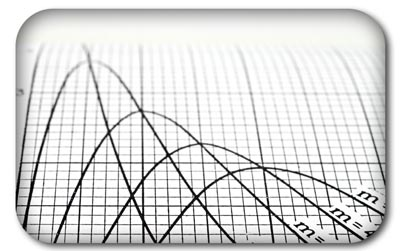# Mathematical Modelling

The development of mechanical engineering systems frequently requires the use of detailed mathematical modelling to optimise performance, integrate active control systems and obtain the required system behaviour.  Dynamiq Engineering provides mathematical modelling services for a variety of typical engineering applications such as:• Performance Modelling
• Component Sizing & Selection
• Dynamic System Design
• Control System Design
• System Identification
• System Optimisation

Models can be provided as mathematical formulations or fully embedded into software form such as Excel Spreadsheets, MATLAB Models, Simulink Models or stand alone applications for Windows or Linux.

Where appropriate models can be formulated using methods defined in relevant standards such as British Standards, ISO, ESDU, UNECE, ASME, ACSE or various Military codes.  Alternatively, Dynamiq is able to draw upon its cross-industry experience to help derive rigorous mathematical models appropriate for specific applications.

Performance Estimation | Component Sizing | Engineering Consulting | Control System Design